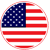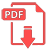# Home | Mathematics | Trigonometry

## Calculate trigonometric values

In pre-calculus, you need to evaluate the six trig functions — sine, cosine, tangent, cosecant, secant, and cotangent — for a single angle on the unit circle. For each angle on the unit circle, three other angles have similar trig function values. The only difference is that the signs of these values are opposite, depending on which quadrant the angle is in. Sometimes the angle won’t be on the unit circle and you’ll have to use your calculator.

If you don’t have the unit circle at your disposal (if you’re taking a test, for instance), you can draw a picture and find the values you need the long way.English